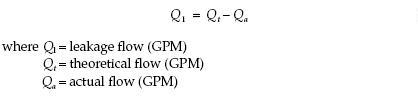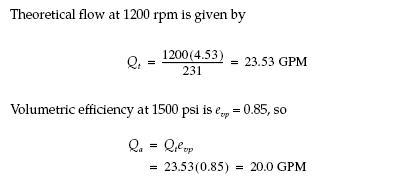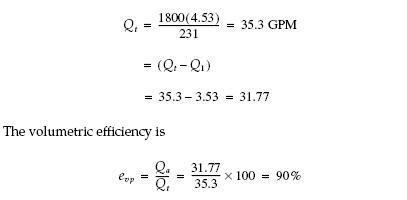﻿ Hydraulic Gerotor Pump Volumetric Efficiency Characteristic – Hydraulic Schematic Troubleshooting

## Hydraulic Gerotor Pump Volumetric Efficiency Characteristic

Hydreco Model 1919 is a gerotor hydraulic pump with 4.53 in3/rev displacement. The pump has maximum speed of 3000 rpm with 2500 psi maximum pressure.

The pump volumetric efficiency drops from 92% at 500 psi to 78% at 2500 psi when the pump rotates at 1200 rpm but at 1800 rpm the volumetric efficiency drops from 93% at 500 psi to 84% at 2500 psi. The gerotor pump volumetric efficiency is higher as the speed increase.

The raising of volumetric efficiency mystery can be unfolded by comparing performance at a specific pressure. The leakage flow at 1500 psi isLeakage flow is a function of pressure, while the rotational speed is giving less significant factor. Suppose that leakage flow is the same for the performance test run at 1800 rpm, 1500 psi.The measured volumetric efficiency was evp = 88.7%, slightly lower than calculated value by assuming constant leakage flow. There is some leakage increasing at higher speeds due to the increased fluid turbulence. This explains why the volumetric efficiency is less than that calculated by assuming constant leakage flow as speed increases. The best design practice is to select a smaller pump and operate it at higher speed to achieve a higher volumetric efficiency.

A gerotor pump can achieve 85 to 90% volumetric efficiency if the working pressures are less than 1500 psi. However, the volumetric efficiency decreases as operating temperature increases. If the temperature increases, the oil viscosity decrease and more leakage occur through the pump clearances.